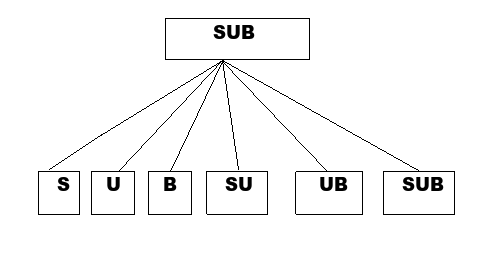# Find the Number of Substrings of a String using C++

In this article, you will learn about the approaches to find the number of substrings (non-empty) that you can form in a given string.Input : string = “moon”
Output : 10
Explanation: Substrings are ‘m’, ‘o’, ‘o’, ‘n’, ‘mo’, ‘oo’, ‘on’, ‘moo’, ‘oon’ and ‘moon’.

Input : string = “yellow”
Output : 21

## Approach to find The Solution

Let the length of the string be n, so looking at the example above, we understand that to find all possible numbers of substrings, we need to add substrings of length n, (n-1), (n-2), (n-3), (n-4),......2, 1.

Total number of substrings = n + (n - 1) + (n - 2) + (n - 3) + (n - 4) + ……. + 2 + 1.

= n * (n + 1) / 2

So now we have a formula for evaluating the number of substrings where n is the length of a given string.

## C++ Code for the Above Approach

Here is the C++ syntax which we can use as an input to solve the given problem −

## Example

#include<bits/stdc++.h>
using namespace std;
int main () {
string str = "yellow";
// finding the length of string
int n = str.length ();
// calculating result from formula
int number_of_strings = n * (n + 1) / 2;
cout << "Number of substrings of a string : " << number_of_strings;
return 0;
}

## Output

Number of substrings of a string: 21

## Explanation of the Code

This is the optimistic and straightforward approach of finding the number of possible substrings from a given string.

Firstly, in this code, we find the length of a given string from the .length() function and put that value in the formula we derived above, printing the output stored in the result variable.

## Conclusion

In this article, we have explained the way to find the number of substrings in a string where we first derive the formula for finding the number of all possible substrings and get the result using the formula from the length of the string. We can write the same program in other languages such as C, java, python, and other languages. We hope you find this article helpful.